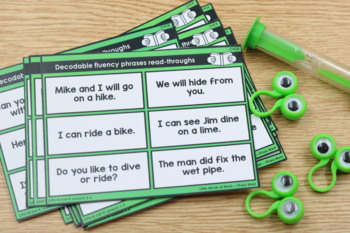Rated 4.91 out of 5, based on 539 reviews
539 Ratings;
K - 2nd, Homeschool
Subjects
Resource Type
Formats Included
• Zip
Pages
75 pages
\$8.00
List Price:
\$16.00
You Save:
\$8.00
Bundle
\$8.00
List Price:
\$16.00
You Save:
\$8.00
Bundle
Report this resource to TPT

#### What educators are saying

Great practice for automatic decoding and fluency. Plenty of practice and easy prep too. The kids enjoyed using them, and I'm already seeing progress.
I love these quick reading checks, especially for back to school. I am able to stop by a kiddos desk and have different starting points to quickly assess where they may need to be placed.

### Description

SNAG THIS BUNDLE ON SALE! EACH PACKET IS VALUED AT \$4 FOR A TOTAL OF \$20!

The Decodable Fluency Phrases Read-Throughs are designed to give students a hands-on and engaging way to practice decodable fluency. This bundle offers 48 decodable fluency phrases read-throughs to practice with via 5 units (cvc, blends/digraphs, cvce, ending blends/r-control, and vowel teams/diphthongs). Each packet covers a systematic scope and sequence which can be found typed below. These decodable fluency phrases read-throughs can be used for whole-group instruction, morning work, early finishers, for your small-group reading instruction, intervention blocks, and of course as an independent literacy center. A printer friendly b/w version is included as well.

ALIGNED TO GUIDED PHONICS + BEYOND CURRICULUM SCOPE!

* * * Questions? Click HERE to Instant Message me! * * *

INCLUDED PACKETS:

Please view the TPT preview to see a detailed look at what is included!

WHAT IS INCLUDED:

• 240 decodable fluency phrases read-through task cards (6 phrases on each card- that's a total of 1,440 phrases!)
• labels

CVC WORDS
Lesson 1: a (/ă/), m (/m/), s (/s/)
Lesson 2: t (/t/)
Lesson 3: p (/p/)
Lesson 4: f (/f/)
Lesson 5: d (/d/)
Lesson 6: i (/ĭ/)
Lesson 7: n (/n/)
Lesson 8: o (/ŏ/)
Lesson 9: c (/k/)
Lesson 10: k (/k/)
Lesson 11: u (/ŭ/)
Lesson 12: ck (/k/)
Lesson 13: b (/b/)

Lesson 14: g (/g/)
Lesson 15: e (/ĕ/)
Lesson 16: h (/h/)
Lesson 17: s (/z/)
Lesson 18: r (/r/)
Lesson 19: l (/l/)
Lesson 20: w (/w/)
Lesson 21: j (/j/)
Lesson 22: y (/y/)
Lesson 23: x (/ks/)
Lesson 24: qu (/kw/), v (/v/)
Lesson 25: z (/z/)

Digraphs and Beginning Blends
Lesson 1: sh (/sh/)
Lesson 2: sh (/sh/)
Lesson 3: th (/th/)
Lesson 4: th (/th/)
Lesson 5: sh (/sh/), th (/th/)
Lesson 6: c, k, ck (/k/)

Lesson 7: ch (/ch/)

Lesson 8: sh (/sh/), th (/th/), ch (/ch/)

Lesson 9: w, wh (/w/)

Lesson 10: sh, th, ck, ch, wh review

Lesson 11: doubled l, f, s, z

Lesson 12: doubled l, f, s, z

Lesson 13: doubled l, f, s, z

Lesson 14: open syllables

Lesson 15: open syllables

Lesson 16: bl-, cl-, fl-

Lesson 17: gl-, pl-, sl-

Lesson 18: l blends review

Lesson 19: cr-, br-, dr-, fr-

Lesson 20: gr-, pr-, tr-

Lesson 21: r blends review

Lesson 22: r blends review

Lesson 23: sc-, sk-, sn-, sm-

Lesson 24: sp-, st-, sw-, tw-

Lesson 25: blends review

CVCE Words
Lesson 1: a_e /ā/
Lesson 2: a_e /ā/
Lesson 3: a_e /ā/
Lesson 4: a (/ă/), a_e (/ā/)
Lesson 5: i_e (/ī/)
Lesson 6: i_e (/ī/)

Lesson 7: i (/ĭ/), i_e (/ī/)

Lesson 8: a_e (/ā/), i_e (/ī/)

Lesson 9: o_e (/ō/)

Lesson 10: o_e (/ō/)

Lesson 11: o (/ŏ/), o_e (/ō/)

Lesson 12: a_e, i_e, o_e

Lesson 13: u_e (/ū/)

Lesson 14: u_e (/ū/)

Lesson 15: u (/ŭ/), u_e (/ū/)

Lesson 16: a_e, i_e, o_e, u_e

Lesson 17: e (/ē/)

Lesson 18: a_e, i_e, o_e, u_e, e_e

Lesson 19: a_e, i_e, o_e, u_e, e_e

Lesson 20: c (/s/)

Lesson 21: c, s, ss (/s/), c (/k/)

Lesson 22: g (/j/)

Lesson 23: j, g (/j/), g (/g/)

Lesson 24: hard and soft review

Lesson 25: y (/ī/)

Ending Blends + R-Control Words
Lesson 1: -ft, -ld, -lp
Lesson 2: -lf, -lk, -lt
Lesson 3: -nd, -nt, -mp
Lesson 4: -sk, -st, -sp
Lesson 5: -pt, -lm, -ct, -xt
Lesson 6: ending -all

Lesson 7: endings -ull, -oll

Lesson 8: endings -nk

Lesson 9: endings -ng

Lesson 10: ar

Lesson 11: or

Lesson 12: er

Lesson 13: ir

Lesson 14: ur, ure

Lesson 15: oar (roar), oor (door)

Lesson 16: our (pour), ore (chore)

Lesson 17: air (fair), ear (pear), are (rare)

Lesson 18: eer (deer), ear (rear)

Lesson 19: r-controlled review

Lesson 20: schwas

Lesson 21: schwas

Lesson 22: kn, gn (/n/)

Lesson 23: wr (/r/), mb (/m/)

Lesson 24: ph (/f/)

Lesson 25: silent sounds review

Vowel Pairs and Diphthongs
Lesson 1: ay (/ā/)
Lesson 2: ai (/ā/)
Lesson 3: ea (/ā/)
Lesson 4: ay, ai, ea, eigh (/ā/)
Lesson 5: ee (/ē/)
Lesson 6: ea (/ē/)

Lesson 7: ey, y (/ē/)

Lesson 8: ee, ea, ey, y (/ē/)

Lesson 9: oa (/ō/)

Lesson 10: ow (/ō/)

Lesson 11: oa, ow, oe (/ō/)

Lesson 12: ie (/ī/)

Lesson 13: y, ie, igh (/ī/)

Lesson 14: ui (/ū/)

Lesson 15: ue (/ū/)

Lesson 16: ui, ue (/ū/)

Lesson 17: au, augh

Lesson 18: aw

Lesson 19: oi

Lesson 20: oy

Lesson 21: ought

Lesson 22: ou, ow

Lesson 23: ew

Lesson 24: oo

Lesson 25: oo, u (/ŏŏ/)

Questions?

Q: Is this bundle included in any other bundle?

A: This bundle is not part of any other bundle in my TPT shop.

Would you like to receive notifications for my newly released packets and upcoming sale? Be sure to FOLLOW ME here on TPT!

**Join my private Facebook Group to join fellow Pre-K, Kindergarten, First Grade and Second Grade teachers that use my curriculum! Collaborate, chat and share ideas with like-minded teachers! Join the private Facebook group H E R E!

Visit my blog Little Minds at Work

Total Pages
75 pages